# How to Multiply, Divide, Simplify Rational Expressions Examples With Solutions

How to multiply, divide and simplify rational expressions? Grade 11 examples are presented along with detailed solutions and more questions with detailed Solutions and explanations are included.
If you have difficulties in multiplying, dividing and simplifying fractions and rational expressions, this tutorial will help you overcome those difficulties on the condition that you understand each step involved in solving these questions and also spend more time practicing if needed. I will present the examples below with more challenging questions as you walk through the tutorial. You need to understand each step!

An online calculator to simplify rational expressions is included and may be used to check results.

## How to multiply, divide and simplify rational expressions?

We multiply two rational expressions by multiplying their numerators and denominators as follows:
1)We divide two rational expressions by multiplying the first rational expression by the reciprocal of the second rational expression as follows:
2)### Example 1

Multiply and simplify:Solution:
Apply the multiplication rule (see above)Factor if possible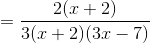Simplify if possible### Example 2

Multiply and simplify:Solution:
Apply the multiplication rule.Factor if possibleSimplify if possible### Example 3

Multiply and simplify: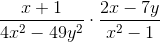.
Solution:
Multiply numerators and denominators (multiplication rule)Factor the two terms in the denominator: 4 x 2 - 49 y 2 = (2x -7y)(2x + 7y) and x 2 - 1 = (x - 1)(x + 1).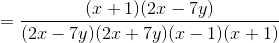Simplify if possible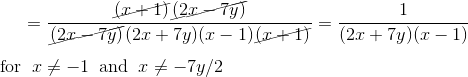### Example 4

Divide and simplify:.
Solution:
The division of two rational expressions is done by multiplying the first rational expression by the reciprocal of the second rational expression as follows (see divison rule above). HenceMultiply numerators and denominators (multiplication rule) but do not expand as we might be able to simplifty.Simplify if possible### Example 5

Divide and simplify: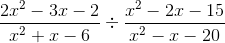.
Solution:
The division of two rational expressions is done by multiplying the first by the reciprocal of the second as follows (see divison rule above). HenceMultiply numerators and denominators (multiplication rule) but do not expand.Factor the terms included in the numerator and denominator (if possible):and use the factored form in the rational expression to simplify### Example 6

Divide and simplify:.
Solution:
We first convert (x - 2) into a rational expression. HenceThe division of two rational expressions is done by multiplying the first by the reciprocal of the second as follows (see divison rule above). HenceMultiply numerators and denominators (multiplication rule) but do not expand.Factor the terms - 2 x + 4 included in the numerator as follows:
- 2 x + 4 = -2(x - 2)
and use - 2 x + 4 in factored form in the rational expression to simplify### More Questions: Multiply and/or divide and simplify the given rational expressions

Detailed
Solutions and explanations to these questions.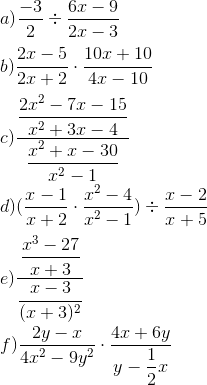Detailed
Solutions and explanations to these questions.